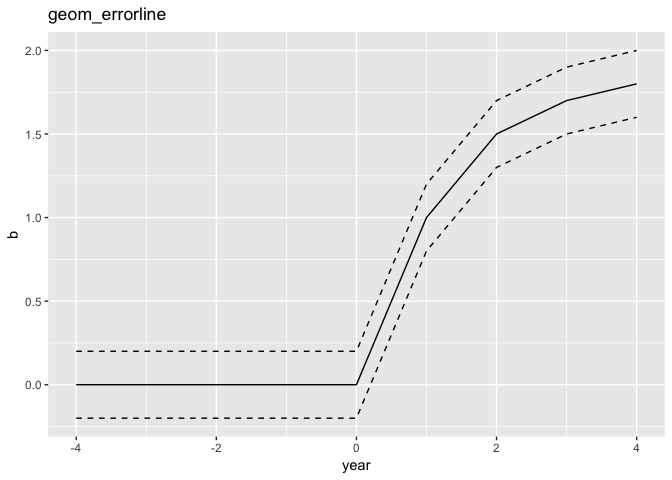{{ message }}
/ ggplot2 Public

# new geom suggestion: separate lines for upper and lower intervals#3503

Closed
opened this issue Aug 25, 2019 · 7 comments · Fixed by #3529
Closed

# new geom suggestion: separate lines for upper and lower intervals#3503

opened this issue Aug 25, 2019 · 7 comments · Fixed by #3529

###weiyangtham commented Aug 25, 2019

 I'm wondering if there's room for a new geom that can produce the following graph ```library(tidyverse) # Example data df <- tibble(y = c(0, 0, 0, 0, 0, 1, 1.5, 1.7, 1.8), upper_y = y + 0.2, lower_y = y - 0.2, year = -4:4) p <- ggplot(df, aes(year, y)) + geom_line() # geom_errorline would simplify this p + geom_line(aes(y = upper_y), linetype = 2) + geom_line(aes(y = lower_y), linetype = 2) ```So instead of having to use `geom_line` twice, you could instead do ```ggplot(df, aes(year, y, ymax = upper_y, ymin = lower_y)) + geom_errorline() ``` and this would fit more neatly into the idea of mapping the upper and lower intervals to the `ymin` and `ymax` aesthetics. I imagine there's a way to achieve that same plot with `geom_ribbon` or `geom_area` but I haven't been able to do so so I'm guessing it wouldn't be straightforward even if there is. Here is a prototype I wrote a few years ago: https://github.com/weiyangtham/econothemes Thanks! The text was updated successfully, but these errors were encountered:

###yutannihilation commented Aug 29, 2019 • edited

 I imagine there's a way to achieve that same plot with `geom_ribbon` or `geom_area` but I haven't been able to do so so I'm guessing it wouldn't be straightforward even if there is. I agree with you. I don't come up with some nice idea for now, but I don't think we need a new geom as this won't be so difficult. e.g. ```library(ggplot2) # Example data df <- tibble::tibble( y = c(0, 0, 0, 0, 0, 1, 1.5, 1.7, 1.8), upper_y = y + 0.2, lower_y = y - 0.2, year = -4:4 ) StatErrorLine <- ggproto("StatErrorLine", Stat, required_aes = c("x", "ymin", "ymax"), default_aes = aes(y = stat(y)), compute_group = function(data, scales) { idx <- names(data) %in% c("y", "ymin", "ymax") data_min <- data_max <- data[!idx] data_min\$y <- data\$ymin data_min\$group <- paste0(data\$group, "-min") data_max\$y <- data\$ymax data_max\$group <- paste0(data\$group, "-max") rbind(data_min, data_max) } ) p <- ggplot(df, aes(year, y)) + geom_line() p + geom_line(aes(ymax = upper_y, ymin = lower_y), linetype = 2, stat = StatErrorLine)```Created on 2019-08-29 by the reprex package (v0.3.0)

###weiyangtham commented Aug 30, 2019 • edited

 I'd thought of it as a geom since I was thinking the more common use case would be one where the upper/lower bounds are provided in the data (e.g. confidence intervals from a model that has been passed through `broom::tidy(model, conf.int = TRUE)`) rather than being a transformation. It would also be more directly analogous to the other "interval" geoms like `geom_errorbar` or `geom_ribbon`, and therefore maybe more intuitive?

###yutannihilation commented Sep 2, 2019

 Now I come to wonder if we can just write this with `geom_ribbon()`: ```ggplot(df, aes(year, y)) + geom_line() + geom_ribbon(aes(ymax = upper_y, ymin = lower_y), linetype = 2, fill = NA, colour = "black")``` I mean, it's just an implementational detail that `GeomRibbon` draws polygons, and it might be able to draw lines and polygons separately. For example, 017992b: ```devtools::load_all("~/Documents/repo/ggplot2") #> Loading ggplot2 df <- tibble::tibble( y = c(0, 0, 0, 0, 0, 1, 1.5, 1.7, 1.8), upper_y = y + 0.2, lower_y = y - 0.2, year = -4:4 ) # can divide the ribbon df[4, "upper_y"] <- NA df[4, "lower_y"] <- NA ggplot(df, aes(year, y)) + geom_line() + geom_ribbon(aes(ymax = upper_y, ymin = lower_y), linetype = 2, fill = "red", alpha = 0.1, colour = "black")```Created on 2019-09-02 by the reprex package (v0.3.0) I'm not sure if this really will be an acceptable change, though...

###weiyangtham commented Sep 4, 2019

 Something like this would be great. Is the worry that users might expect `geom_ribbon()` to always draw polygons?

###yutannihilation commented Sep 5, 2019

 users might expect `geom_ribbon()` to always draw polygons? I expected there's effectively no such users, but I'm not fully sure... Just curious, have you ever seen such a usage?

###weiyangtham commented Sep 5, 2019

 Just to make sure we're on the same page, are you asking if I've seen someone use `geom_ribbon` with a transparent fill but they still want the polygon? I don't think I've seen that before.

###yutannihilation commented Sep 5, 2019

 Yes, that's what I meant to ask. Thanks.